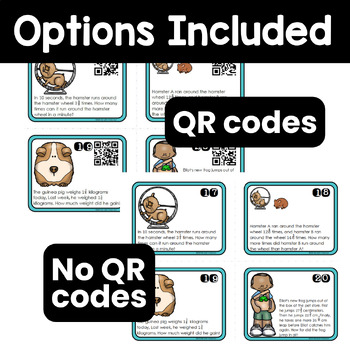4th - 5th, Homeschool
Subjects
Standards
Resource Type
Formats Included
• PDF
Pages
24 pages

Description

Fraction practice! This is a set of 32 word problem task cards in a pet shop theme. Use for end of year 4th grade review, test prep, or beginning of 5th grade review/assessment. Feel free to add them to your math centers, for a word problem of the day in your math journals, or even a game of Scoot! An answer key and recording sheet are provided.

These problems can be used to support the following Common Core State Standards:

4.NF.A.2, 4.NF.B.3a, 4.NF.B.3b, 4.NF.B.3c, 4.NF.B.3d, 4.NF.B.4a, 4.NF.B.4, 4.NF.C.5, 5.NF.A.1, 5.NF.A.2, 5.NF.B.6, 5.NF.B.7c

**Please note there is a duplicate set of these word problems without the QR codes if you don't have a smartphone/tablet at your disposal. There is also an answer key and recording sheet provided (black and white version also included). If you are using the QR codes, please keep in mind that they can be read from a computer screen as well so if that is an option for your classroom, you can save yourself paper and ink that way!

You may also want to download these complementary FREE resources to support word problem solving:

Word Problem Guide: Steps, FREE
Math Clue Words Posters FREE

•••••••••••••••••••••••••••••••••••••••••••••••••••••••••••••••••••••••••••••••••
Word Problem Task Cards Operations & Algebraic Thinking, 4th Grade (QR Codes)
Word Problem Task Cards Measurement and Data, 4th Grade (QR Codes) Common Core
Word Problems Task Cards Numbers & Operations in Base Ten, 4th Grade (QR Codes)
Elapsed Time Task Cards (Optional QR Codes) 3rd / 4th Grade Common Core
Simplifying Fractions Task Cards: Simplify, Simplify, Compare (QR Code Option)
•••••••••••••••••••••••••••••••••••••••••••••••••••••••••••••••••••••••••••••••••

Total Pages
24 pages
Included
Teaching Duration
2 hours
Report this Resource to TpT
Reported resources will be reviewed by our team. Report this resource to let us know if this resource violates TpT’s content guidelines.

Standards

to see state-specific standards (only available in the US).
Express a fraction with denominator 10 as an equivalent fraction with denominator 100, and use this technique to add two fractions with respective denominators 10 and 100. For example, express 3/10 as 30/100, and add 3/10 + 4/100 = 34/100.
Solve real world problems involving division of unit fractions by non-zero whole numbers and division of whole numbers by unit fractions, e.g., by using visual fraction models and equations to represent the problem. For example, how much chocolate will each person get if 3 people share 1/2 lb of chocolate equally? How many 1/3-cup servings are in 2 cups of raisins?
Solve real world problems involving multiplication of fractions and mixed numbers, e.g., by using visual fraction models or equations to represent the problem.
Solve word problems involving addition and subtraction of fractions referring to the same whole, including cases of unlike denominators, e.g., by using visual fraction models or equations to represent the problem. Use benchmark fractions and number sense of fractions to estimate mentally and assess the reasonableness of answers. For example, recognize an incorrect result 2/5 + 1/2 = 3/7, by observing that 3/7 < 1/2.
Add and subtract fractions with unlike denominators (including mixed numbers) by replacing given fractions with equivalent fractions in such a way as to produce an equivalent sum or difference of fractions with like denominators. For example, 2/3 + 5/4 = 8/12 + 15/12 = 23/12. (In general, 𝘢/𝘣 + 𝘤/𝘥 = (𝘢𝘥 + 𝘣𝘤)/𝘣𝘥.)